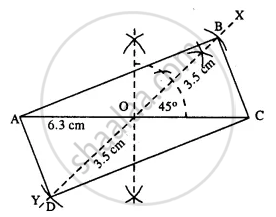# Construct a Parallelogram Abcd, If : Lengths of Diagonals Ac and Bd Are 6.3 Cm and 7.0 Cm Respectively, and the Angle Between Them is 45°. - Mathematics

Sum

Construct a parallelogram ABCD, if :

lengths of diagonals AC and BD are 6.3 cm and 7.0 cm respectively, and the angle between them is 45°.

#### SolutionSteps :

1. Draw AC = 6.3 cm.
2. Bisect AC at O.
3. At O, draw ∠XOC = 45° and produce XO to Y.
4. Cut OB = OD = 3.5 cm (half the diagonal 7 cm.)
5. Join AB, CB, AD, and CD. Thus ABCD is the required || gm.
Concept: Construction of Parallelograms
Is there an error in this question or solution?

#### APPEARS IN

Selina Concise Mathematics Class 8 ICSE
Chapter 18 Constructions
Exercise 18 (D) | Q 2.6 | Page 211